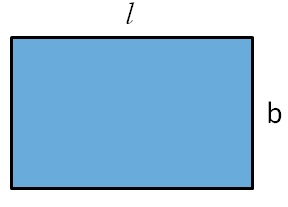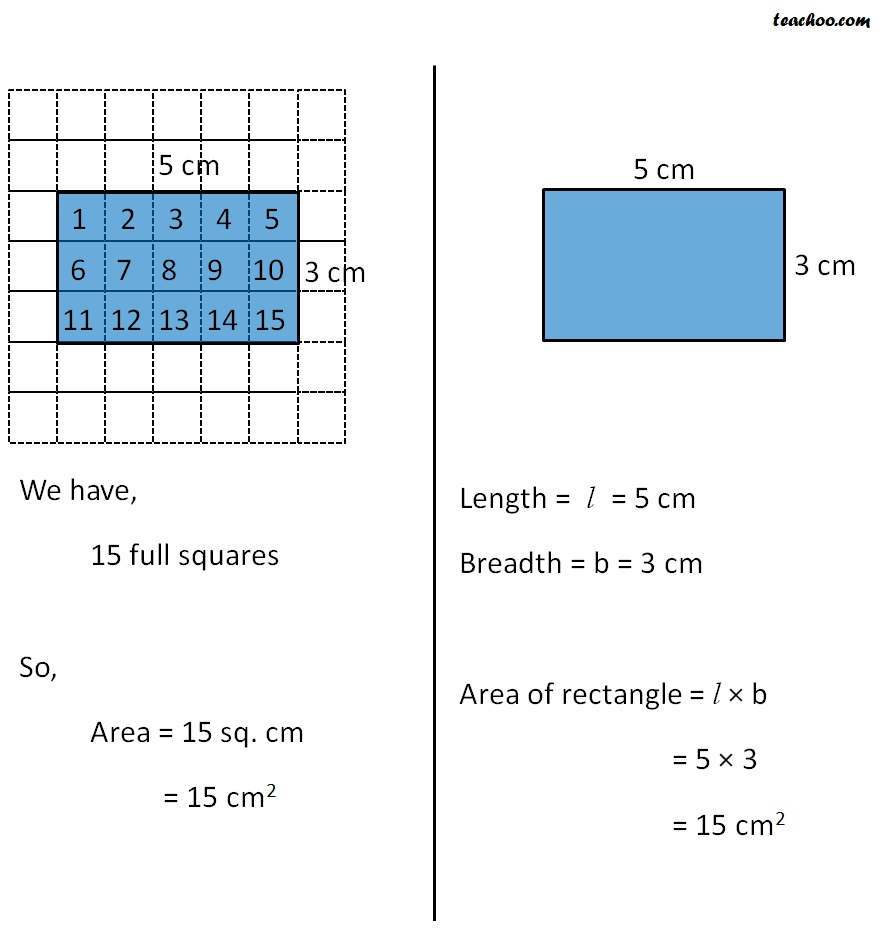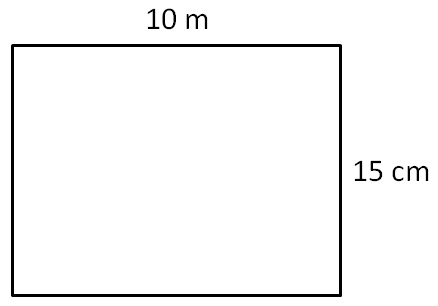We had found area of rectangle by counting the squares

But, that method is too long

To find area of any rectangle,

We use formula

For a rectangle with length l and breadth bArea of rectangle = l × b

#### Comparing counting the squares method to formula#### Find area of rectangle with length 10 m and breadth 15 mLength = l = 10 m

Breadth = b = 15 m

Area of rectangle = l × b

= 10 × 15

= 150 sq. m

= 150 m 2

1. Chapter 11 Class 7 Perimeter and Area
2. Concept wise
3. Square and Rectangle

Transcript

We have, 15 full squares So, Area = 15 sq. cm = 15 cm2 Length = l = 5 cm Breadth = b = 3 cm Area of rectangle = l × b = 5 × 3 = 15 cm2

Square and Rectangle

About the AuthorDavneet Singh
Davneet Singh is a graduate from Indian Institute of Technology, Kanpur. He has been teaching from the past 9 years. He provides courses for Maths and Science at Teachoo.